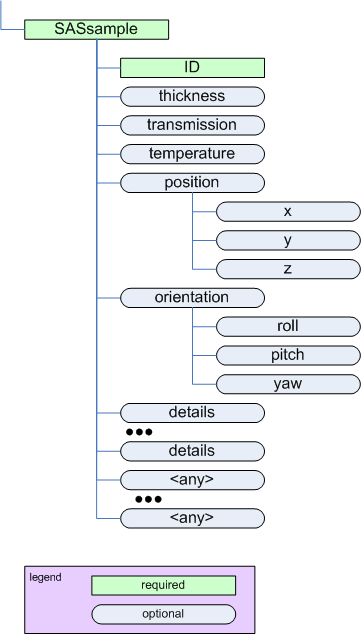#### Previous topic

SAStransmission_spectrum

SASinstrument

# SASsample¶

parent:
SASentryThe SASsample element

Name Type Occurrence Description Attributes
ID string [1..1] Text string that identifies this sample.
thickness float [0..1] Thickness of this sample. unit={unit} 
transmission float [0..1] Transmission ($$I/I_0$$) of this sample. Note that there is no unit attribute as this number is dimensionless.
temperature float [0..1] Temperature of this sample. unit={unit} 
position container [0..1] Location of the sample in X, Y, and Z.
orientation container [0..1] Orientation (rotation) of the sample.
details string [0..inf] Any additional sample details.
{any} container [0..inf] Any element(s) not defined in the cansas1d:1.1 standard can be placed at this point. xmlns:{foreign-prefix}={foreign-namespace}

## geometry¶

See the figures in Definition of the coordinate axes.

## position¶

Name Type Occurrence Description Attributes
$$x$$ float [0..1] Position of the sample in X. unit={unit} 
$$y$$ float [0..1] Position of the sample in Y. unit={unit} 
$$z$$ float [0..1] Position of the sample in Z. unit={unit}  

## orientation¶

Note

The orientation element is intended to describe simple rotations about a single axis rather than a full set of rotations as in a crystallographic context.

Name Type Occurrence Description Attributes
roll float [0..1] Rotation about the Z axis (roll). unit={unit} 
pitch float [0..1] Rotation about the X axis (pitch). unit={unit} 
yaw float [0..1] Rotation about the Y axis (yaw). unit={unit} 

Table Notes

  (1, 2, 3, 4, 5, 6, 7, 8) The unit attribute is required. See Rules for acceptable values.
  While $$z$$ is allowed by the standard (provided by use of a standard size element in the XML Schema), it does not make sense to use it for small-angle scattering in some situations as noted. Use of $$z$$ in such situations may be ignored by processing software.